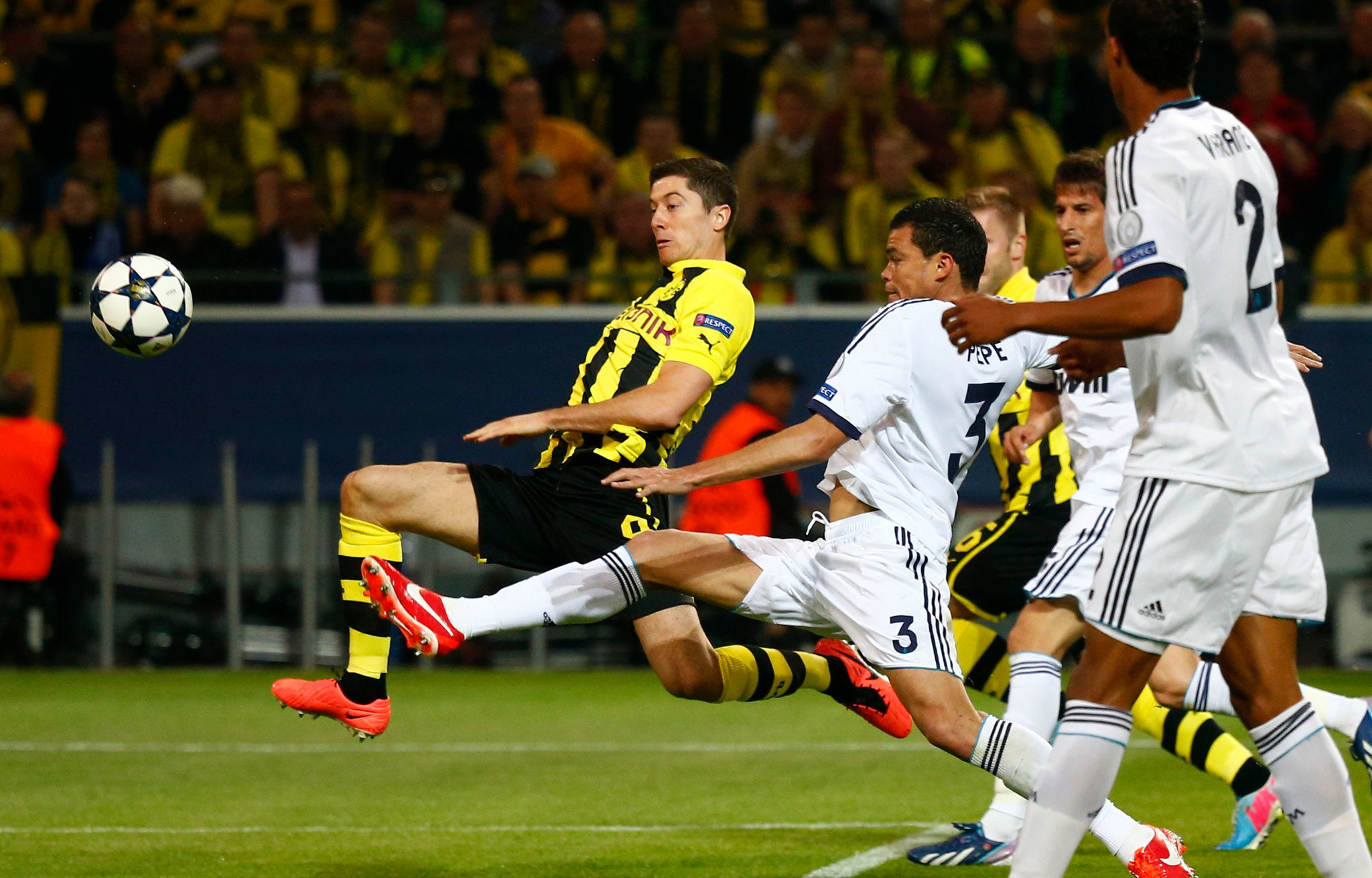# How to secure your predictions in sports betting?

It is essential to know how to secure your predictions. There are several methods to do this.

• Double Luck.
• The “Payback if” Bet.

And we will give you the mathematical explanation of both types of predictions so that you can make them manually and scratch on the margin that bookmakers make on your back.

## TERMS USED FOR DOUBLE CHANCE

• +1: Win or draw
• +2: Does not lose or loses by 1
• +3: Does not lose or loses by 2

Double chance bookmaker

With the operator, the hopes of gains would be:

• 1/X: 100*2.08 = 208
• 1/2: 100*1.18 = 118
• X/2: 100*1.10 = 110

Done manually, the hopes of earnings would be:

Formula: Total stake * (odds A * odds B)/(odds A + odds B) = Double chance winnings

• 1/X: 100*(6.25*3.9)/(6.25+3.9) = 100*2.4014 = 240.14
• 1/2: 100*(6.25*1.57)/(6.25+1.57) = 100*1.2547 = 125.47
• X/2: 100*(3.9*1.57)/(3.9+1.57) = 100*1.1193 = 111.93

It can therefore be seen that the manual winnings are higher than the operator’s winnings (be careful, it sometimes happens that the operator’s double chance is higher than the manual, this is particularly the case during “+2”, i.e. such and such a team wins, draws or loses by 1).

To know the bet on each team in the case of the manual hypothesis, the hope of winning must be divided by the double chance odds:

For the 1/X:

• bet on 1: 240.14/6.25 = 38.42
• put on the X: 240.14/3.9 = 61.58

For the 1/2:

• put on the 1: 125.47/6.25 = 20.08
• put on the 2: 125.47/1.57 = 79.92

For the X/2:

• put on the X: 111.93/3.9 = 28.7
• put on the 2: 111.93/1.57 = 71.3

## TERMS USED FOR “REIMBURSED IF” PROGNOSES

ANB: Away no Bet = refunded if away win

DNB: Draw no Bet = refunded if match is drawn

HNB: Home no Bet = reimbursed if you win at home

## CONCRETE EXAMPLE

For the match Apollon Limassol – Lyon, here are the odds proposed by a Bookmaker.

We will consider that the bet for this match will be 100 €.

### Event odds

Here is an example of what is called a “Draw No Bet”, entitled “Draw No Bet” on your online betting sites.

### DNB bookmaker

With the operator, the hopes of gains would be:

• 1 except for a draw: 100*3.50 = 350
• 2 out of a draw: 100*1.14 = 114

Done manually, the hopes of earnings would be:

Formula: (Total stake – (Total stake / odds of the envisaged refund)) * odds of the hypothesis considered = Winnings of the bet reimbursed.

• 1 excluding draw: (100-(100/3.9))*6.25 = 464.74
• 2 except for a draw: (100-(100/3.9))*1.57 = 116.74

It can be seen that the manual winnings are higher than the operator’s proposed winnings also in the case of the refunded bet. But also that the operators do not offer a win-less result at home (HNB) or a win-less result away (ANB).

To know the bet on each team in the case of the manual hypothesis, it is necessary:

Formula: Bet on the hypothesis considered = Total bet – Bet on the refund with Repayment stake = Total stake / Score of the envisaged repayment.

For DNB home victory:

• put on the X: 100/3.9 = 25.64 (13)
• put on 1: 100-25.64 = 74.36

For the victory outside DNB:

• put on the X: 100/3.9 = 25.64
• put on 2: 100-25.64 = 74.36

## SECURE YOUR PREDICTIONS MANUALLY WITH OUR TOOLS

We wanted to make your life easier by providing you with tools for calculating and allocating bids:

• Calculation Paris “reimbursed if”.
• Double Chance Calculation.

### Related Posts